# Applications of Derivatives Set 11

Go back to  'SOLVED EXAMPLES'

Example - 25

Plot the graphs of the following functions:

 $$(a)\quad f\left( x \right) = 2\sin x + \cos 2x$$ (b)\quad \begin{align}f\left( x \right) = 3x + \frac{{3x}}{{x - 1}}\end{align} (c) \quad \begin{align}f\left( x \right) = \frac{{3x}}{2}\ln \left( {e - \frac{1}{{3x}}} \right)\end{align} (d)\begin{align}\quad f\left( x \right) = \sqrt {1 + {x^2}} \sin \frac{1}{x}\end{align} (e)\quad \begin{align}f\left( x \right) = \frac{{2{x^3}}}{{{x^2} - 4}}\end{align} (f)\quad \begin{align}f\left( x \right) = \frac{{\left( {x + 1} \right)}}{{\left( {x - 1} \right)\left( {x - 7} \right)}}\end{align} (g) \begin{align} f\left( x \right) = \frac{x}{{\ln x}}\end{align} $$(h)f\left( x \right) = x\ln x$$

Solution: (a) Observe that f(x) is periodic with period $$2\pi$$. Therefore, we can analyses f(x) only for the interval $$[0,2\pi ],$$and by virtue of its periodicity, this analysis will remain applicable in all intervals of the form$$\left[ {2n\pi ,\left( {2n + 1} \right)\pi } \right],\,\,n\, \in \,\mathbb{Z}$$.

Now,

\begin{align}&f'\left( x \right) = 2\cos x - 2\sin 2x\\\\&\;\,\,\,\,\,\,\,\,\,\,\,\,\, = 2\cos x\left( {1 - 2\sin x} \right)\end{align}

This is 0 when

\begin{align}&\,\,\,\,\,\,\,\,\,\,\,\,\,\,\,\,\,\cos x = 0\,\,\text{or} \,\,1 - 2\sin x = 0\\\\& \Rightarrow \quad x = \frac{\pi }{2},\frac{{3\pi }}{2}\,\,{\text{or}}\,\,x = \frac{\pi }{6},\frac{{5\pi }}{6}\end{align}

Therefore, we have 4 extremum points, namely

$x = \frac{\pi }{6},\,\,\frac{\pi }{2},\,\frac{{5\pi }}{6},\frac{{3\pi }}{2}$

We now evaluate $$f''\left( x \right)$$ at each of these points

\begin{align} &\quad \quad\;\; f''\left( x \right)=-2\sin x-4\cos 2x \\\\ &\quad \quad\;\;f''\left( \frac{\pi }{6} \right)=-2\times \frac{1}{2}-4\times \frac{1}{2}=-3<0 \\\\ & \Rightarrow \quad x=\frac{\pi }{6}\,\text{is a point of local maximum}\,\text{;}f\left( \frac{\pi }{6} \right)=\frac{3}{2} \\\\ &\quad \quad\;\; f''\left( \frac{\pi }{2} \right)=-2\times 1-4\times \left( -1 \right)=2>0 \\\\ & \Rightarrow \quad x=\frac{\pi }{2}\;\,\text{is a point of local minimum;}\,\,f\left( \frac{\pi }{2} \right)=1 \\ \\ &\quad \quad\;\; f''\left( \frac{5\pi }{6} \right)=-2\times \frac{1}{2}-4\frac{1}{2}=-3<0 \\\\ & \Rightarrow\quad x=\frac{5\pi }{6}\,\,\text{is a point of local maximum}\,;f\left( \frac{5\pi }{6} \right)=\frac{3}{2} \\\\ &\quad \quad\;\; f''\left( \frac{3\pi }{2} \right)=\left( -2\times -1 \right)-4\times \left( -1 \right)=6>0 \\ & \Rightarrow \quad x=\frac{3\pi }{2}\,\,\text{is a point of local minimum;}\,\,f\left( \frac{3\pi }{2} \right)=-3 \\ \end{align}

Additionally, f(0) = 1 and$$f\left( {2\pi } \right) = 1$$

The graph is obtained by plotting these points and joining them by a smooth curve; and finally replicating this graph periodically: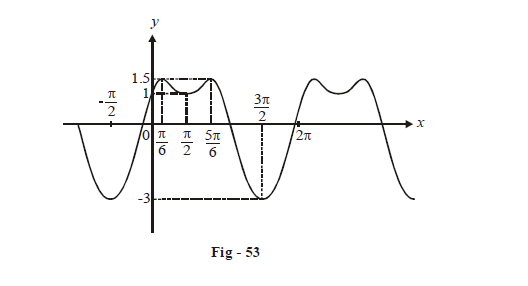(b) Notice that$$\mathop {\lim }\limits_{x \to {1^ - }} f\left( x \right) = - \infty \,\,{\rm{and}}\,\,\mathop {\lim }\limits_{x \to 1 + } f\left( x \right) = + \infty$$

$$\Rightarrow \quad x = 1$$ is an asymptote for the given curve.

Now,

\begin{align}&f'\left( x \right) = 3 + \frac{{3\left( {x - 1} \right) - 3x}}{{{{\left( {x - 1} \right)}^2}}}\\\\&\,\,\,\,\,\,\,\,\,\,\,\,\; = 3 - \frac{3}{{{{\left( {x - 1} \right)}^2}}}\\\\&\,\,\,\,\,\,\,\,\,\,\; = \frac{{3\left( {{x^2} - 2x} \right)}}{{{{\left( {x - 1} \right)}^2}}}\end{align}

This is 0 when x = 0, 2

Therefore, there are two extremum points.

$f\left( 0 \right)=0\,\,\text{and}\,\,f\left( 2 \right)=12$

We need not determine $$f''\left( x \right)$$ here; we just observe that there is another asymptote to this curve. This is how it can be determined:

$\mathop {\lim }\limits_{x \to \pm \infty } \frac{y}{x} = \mathop {\lim }\limits_{x \to \pm \infty } \left( {3 + \frac{3}{{x - 1}}} \right) = 3$

Also,                                                                                      $$\mathop {\lim }\limits_{x \to \pm \infty } \left( {y - 3x} \right) = \mathop {\lim }\limits_{x \to \pm \infty } \left( {\frac{{3x}}{{x - 1}}} \right) = 3$$

Therefore, $$y - 3x = 3$$ is another asymptote to the curve.

We now simply draw the two asymptotes (and deduce that x = 2 must be a minimum point and x = 0 must be a maximum point)

The graph is drawn below; observe the details carefully: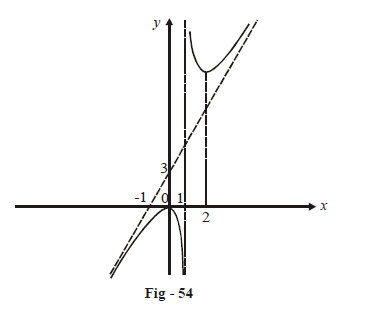(c) The domain of the this function is given by:

\begin{align}{}e - \frac{1}{{3x}} > 0\\ \Rightarrow x < 0\,\,\,{\rm{or}}\,\,x > \,\,\frac{1}{{3e}}\end{align}

Since \begin{align}\mathop {\lim }\limits_{x \to \frac{{{1^ + }}}{{3e}}} f\left( x \right) = - \infty ,\,\,x = \frac{1}{{3e}}\end{align}is an asymptote to the given curve.

Also,$$\mathop {\lim }\limits_{x \to {0^ - }} f\left( x \right) = 0$$

Now,

\begin{align}&\mathop {\lim }\limits_{x \to \pm \infty } \frac{y}{x} = \mathop {\lim }\limits_{x \to \pm \infty } \left( {\frac{3}{2}\ln \left( {e - \frac{1}{{3x}}} \right)} \right) = \frac{3}{2}\ln e = \frac{3}{2}\\\\&\mathop {\lim }\limits_{x \to \pm \infty } \left( {y - \frac{{3x}}{2}} \right) = \frac{3}{2}\mathop {\lim }\limits_{x \to \pm \infty } x\left( {\ln \left( {e - \frac{1}{{3x}}} \right) - 1} \right)\\\\&\qquad\qquad \qquad\quad= \frac{{ - 1}}{{2e}}\,\,\,\,\,\,\,\,\left( {verify} \right)\end{align}

$\Rightarrow \text{ }\!\!~\!\!\text{ }y=\frac{3x}{2}\,-\,\frac{1}{2e}\,\,\text{is another asymptote to the curve}.$

We now simply draw the two asymptotes from which the graphs easily follows.

(notice that we did not even evaluate f'(x); why?).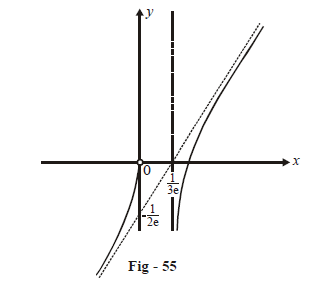(d) As we have seen previously, $$y = \sqrt {1 + {x^2}}$$ will act as an envelope for the given f(x). Around the origin, the oscillations will be extremely fast and will “spread out” away from the origin

Now,

\begin{align} & \begin{gathered} \underset{x\to \infty }{\mathop{\lim }}\,f\left( x \right)=\underset{x\to \infty }{\mathop{\lim }}\,\left( \sqrt{1+{{x}^{2}}}\sin \frac{1}{x} \right) \\ \qquad\qquad\qquad\quad =\underset{x\to \infty }{\mathop{\lim }}\,\left[ \frac{\sqrt{1+{{x}^{2}}}}{x}\cdot \left\{ \frac{\sin \left( \frac{1}{x} \right)}{\frac{1}{x}} \right\} \right] \\ \end{gathered} \\ &\qquad\qquad\qquad\quad=1\\ & \Rightarrow \quad \text{ }\!\!~\!\!\text{ }y=1\,\,\text{is an asymptote to the curve}. \\ \end{align}

Similarly,

$$\mathop {\lim }\limits_{x \to - \infty } f\left( x \right) = - 1$$

$$\Rightarrow y = - 1$$ is also an asymptote to the curve.

The roots of f(x) = 0 are given by:

$\frac{1}{x} = n\pi \,\,;\,\,n \in \mathbb{Z}\,\,\,\,\,\,\,\,\,\,\,\,\,\,\,\,\,\,\,\,\,\,\,\,\,\, \Rightarrow x = \frac{1}{{n\pi }}\,;\,\,n \in \mathbb{Z}$

This information is now sufficient do draw the graph of f(x)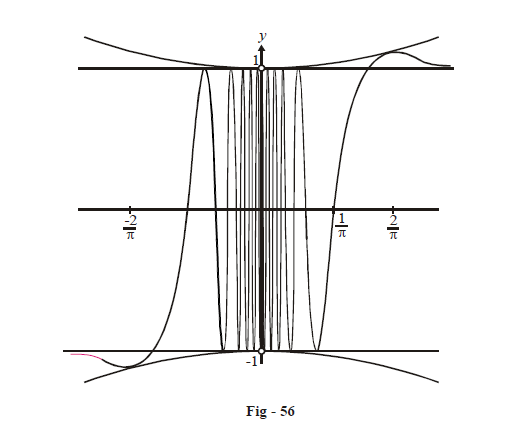(e) The domain of the given function is

$$D = \mathbb{R}\backslash \left\{ { - 2,\,\,2} \right\}$$

$$\Rightarrow \quad x = - 2\,\,{\rm{and}}\,\,x = 2$$ are asymptotes to the curve.

Now we evaluate the essentials limits of (x):

\begin{align}&\mathop {\lim }\limits_{x \to \infty } f\left( x \right) = + \infty \,\,{\rm{and}}\,\,\mathop {\lim }\limits_{x \to - \infty } f\left( x \right) = - \infty \\\\&\mathop {\lim }\limits_{x \to {2^ + }} f\left( x \right) = + \infty \,\,{\rm{and}}\,\,\mathop {\lim }\limits_{x \to {2^ - }} f\left( x \right) = - \infty \\\\&\mathop {\lim }\limits_{x \to - {2^ + }} f\left( x \right) = + \infty \,\,{\rm{and}}\,\,\mathop {\lim }\limits_{x \to - {2^ - }} f\left( x \right) = - \infty \\\\&\mathop {\lim }\limits_{x \to 0} f\left( x \right) = 0\,\,{\rm{and}}\,\,f\left( 0 \right) = 0\\\\&\mathop {\lim }\limits_{x \to \pm \infty } \left( {\frac{y}{x}} \right) = \mathop {\lim }\limits_{x \to \pm \infty } \left( {\frac{{2{x^2}}}{{{x^2} - 4}}} \right) = 2\\\end{align}
$\text{and} \mathop {\lim }\limits_{x \to \pm \infty } \left( {y - 2x} \right) = \mathop {\lim }\limits_{x \to \pm \infty } \left( {\frac{{8x}}{{{x^2} - 4}}} \right) = 0$

$\Rightarrow \quad y = 2x \text{ is another asymptote to the curve.}$

Finally,   \begin{align}f'\left( x \right) = \frac{{2{x^2}\left( {{x^2} - 12} \right)}}{{{{\left( {{x^2} - 4} \right)}^2}}}\end{align}

This is 0 when x = 0$$\pm 2\sqrt 3$$,

While drawing the graph, it becomes obvious that $$x = 2\sqrt 3$$ is a local minimum point while is a local maximum point. The only additional observation we need to make is that $$x = - 2\sqrt 3$$ so that x = 0 is neither a local maximum nor a local minimum but a point of inflexion: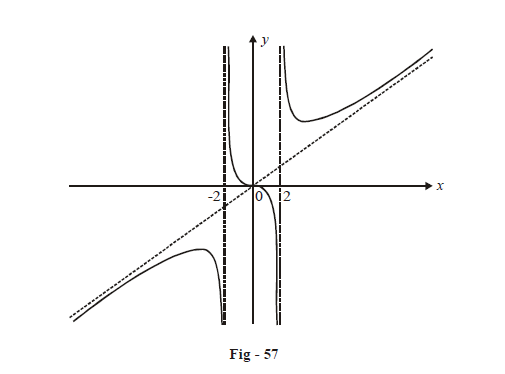(f) The domain of the given function is $$\mathbb{R}\backslash \{ 1,\,\,7\}$$.

$$\Rightarrow x = 1,\,\,x = 7$$ will be asymptotes to the curve.

\begin{align}&\mathop {\lim }\limits_{x \to {7^ + }} f\left( x \right) = + \infty \,\,{\rm{and}}\,\,\mathop {\lim }\limits_{x \to {7^ - }} f\left( x \right) = - \infty \\\\&\mathop {\lim }\limits_{x \to {1^ + }} f\left( x \right) = - \infty \,\,{\rm{and}}\,\,\mathop {\lim }\limits_{x \to {1^ - }} f\left( x \right) = + \infty \end{align}

Also,

$$\mathop {\lim }\limits_{x \to \pm \infty } f\left( x \right) = 0$$

Observe that f(x) has a zero at x = –1.

Also,

$f\left( x \right) > 0\,\,{\rm{for}}\,\,x \in \left( { - 1,\,\,1} \right) \cup \left( {7,\,\,\infty } \right) and$

$f\left( x \right) < 0\,\,{\rm{for}}\,\,x \in \left( { - \infty ,\, - 1} \right) \cup \left( {1,\,\,7} \right)$

Now,\begin{align} f'\left( x \right) = \frac{{\left( {{x^2} - 8x + 7} \right) - \left( {2x - 8} \right)\left( {x + 1} \right)}}{{{{\left\{ {\left( {x - 1} \right)\left( {x - 7} \right)} \right\}}^2}}}\end{align}

\begin{align}= - \frac{{\left( {x + 5} \right)\left( {x - 3} \right)}}{{{{\left( {x - 1} \right)}^2}{{\left( {x - 7} \right)}^2}}}\end{align}

$$\Rightarrow \quad \,f'\left( x \right) > 0\,\,\,\forall \,\,x \in \left( { - 5,\,\,1} \right) \cup \,\,\left( {1,3} \right)$$

or $$f\left( x \right)$$ increases in these intervals

$$\Rightarrow \quad \,f'\left( x \right) < 0\,\,\forall \,\,x\,\, \in \,\,\left( { - \infty , - 5} \right) \cup \left( {3,7} \right) \cup \left( {7,\infty } \right)$$

or $$f\left( x \right)$$ decreases in these intervals.

\begin{align}f( - 5) = \frac{{ - 1}}{{18}},f(3) = \frac{{ - 1}}{2},f(0) = \frac{1}{7}\end{align}

We now have sufficient information to plot the graph accurately: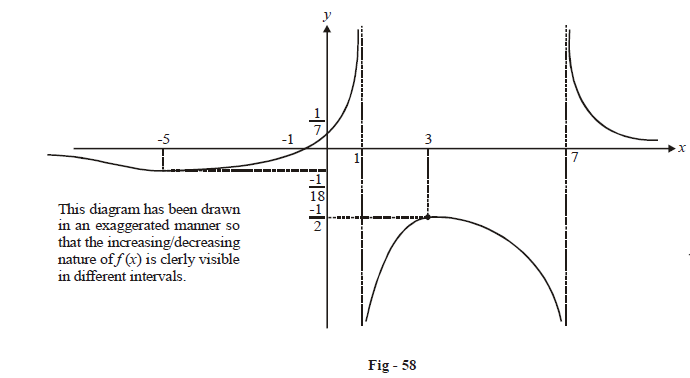(g) The domain of the given function will be $$\left( {0,\,\,\infty } \right)\backslash \left\{ {\,1\,} \right\}$$

\begin{align} \mathop {\lim }\limits_{x \to {1^ + }} \frac{x}{{\ln x}} = + \infty ,\,\,\mathop {\lim }\limits_{x \to {1^ - }} \frac{x}{{\ln x}} = - \infty \end{align}

$$\Rightarrow \,x = 1$$ will be an asymptote to the given curve.

Also,            \begin{align}\mathop {\lim }\limits_{x \to \infty } \frac{x}{{\ln x}} = \mathop {\lim }\limits_{x \to \infty } \frac{1}{{1/x}}\end{align}        (L.H rule)

\begin{align}\mathop {\lim }\limits_{x \to 0} \frac{x}{{\ln x}} = 0\end{align}

Now,   \begin{align}f'\left( x \right) = \frac{{\ln x - 1}}{{{{\left( {\ln x} \right)}^2}}}\end{align}

This is 0 when x = e

\begin{align}& \Rightarrow f'\left( x \right) > 0\,\,{\rm{for}}\,\,x > e\,\,{\rm{or}}\,f\left( x \right)\,{\rm{increases}}\,{\rm{on}}\,\left( {e,\infty } \right)\\\\& \Rightarrow f'\left( x \right) < 0\,\,{\rm{for}}\,\,x < e\,\,{\rm{or}}\,f\left( x \right)\,{\rm{decreases}}\,{\rm{on}}\,\left( {0,e} \right)\backslash \left\{ {\,1\,} \right\}\\\\&\,\,\,\,\,\,\,\,\,f\left( e \right) = e\end{align}

The graph, based on this information, is drawn below.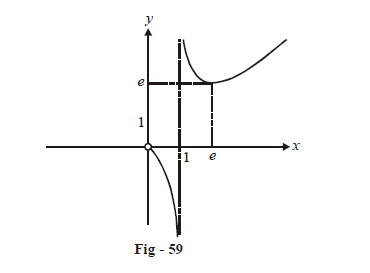(h) The domain of the given function is $$\left( {0,\,\infty } \right)$$

Now,       $$\mathop {\lim }\limits_{x \to 0} x\ln x = 0$$           (we evaluated this in the unit on limits)

$$\mathop {\lim }\limits_{x \to \infty } x\ln x = \infty$$

\begin{align}f'\left( x \right) = 1 + \ln = x\end{align}

This is 0 if \begin{align}x = \frac{1}{e}\end{align}

\begin{align} \Rightarrow f\left( x \right)\end{align} decreases when\begin{align} 1 + \ln x < 0\,\,{\rm{or}}\,\,x \in \left( {0,\frac{1}{e}} \right)\end{align}

and \begin{align}f\left( x \right)\end{align}increases when\begin{align}1 + \ln x > 0\,\,{\rm{or}}\,\,x \in \left( {\frac{1}{e},\infty } \right)\end{align}

\begin{align}f\left( {\frac{1}{e}} \right) = \frac{{ - 1}}{e}\end{align}

Also,     $$f\left( x \right) = 0\,\,{\rm{at}}\,\,x = 1$$

The graph is drawn below based on these calculations: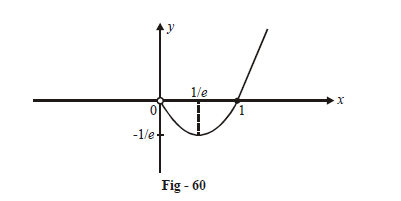Learn from the best math teachers and top your exams

• Live one on one classroom and doubt clearing
• Practice worksheets in and after class for conceptual clarity
• Personalized curriculum to keep up with school